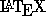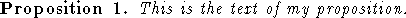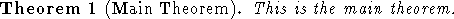Next: Lists Up: Commands for Text Mode Previous: A Word About Environments

## Theorems, Lemmas, and Conjecturesprovides a simple way to typeset the statements of Theorems, Lemmas, Conjectures and so on. For each such type of statement appearing in your text, you create an environment using the \newtheorem command. For example,

\newtheorem{prop}{Proposition}
sets up an environment named prop for typesetting Propositions. After this command has been used, you can type
\begin{prop}
This is the text of my proposition.
\end{prop}
to getNotice that the proposition was automatically numbered and typeset in italics.

A \newtheorem command can go anywhere in the file before the first use of the environment it defines. For simplicity, it is conventional to put it at the front of the file between the \documentstyle line and \begin{document}. (No text is allowed to appear before \begin{document}, but commands like \newtheorem, which generate no output, are permissible.)

You can give a theorem a name, or credit the discoverer, by giving the \begin command an argument in square brackets. For instance,

\newtheorem{thm}{Theorem}
\begin{thm}[Main Theorem]
This is the main theorem.
\end{thm}
producesSee Lamport's book for more details about \newtheorem.

Processed by LaTeX2html